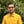Trusted answers to developer questions
Trusted Answers to Developer Questions

Related Tags

swift
sin()
math
communitycreator

# What is the sin() function in Swift?Harris Amjad

Grokking Modern System Design Interview for Engineers & Managers

Ace your System Design Interview and take your career to the next level. Learn to handle the design of applications like Netflix, Quora, Facebook, Uber, and many more in a 45-min interview. Learn the RESHADED framework for architecting web-scale applications by determining requirements, constraints, and assumptions before diving into a step-by-step design process.

### Overview

In Swift, the sin() function returns the sine of a number.

The illustration below shows the mathematical representation of the sin() function:

Mathematical representation of the sin() function

Note: We need to import Foundation in our code to use the sin() function. We can import it like this: import Foundation.

### Syntax

sin(number)
//number can be real, float, or double.


### Parameters

This function requires a number that represents an angle as the parameter.

In order to convert degrees to radians, use the following formula:

radians = degrees * ( pi / 180 )


### Return value

This function returns the sine of the number that is sent as a parameter. The return value will be between -1 and 1.

### Example

import Swiftimport Foundation//positive number in radiansprint("The value of sin(2.3) :", sin(2.3));// negative number in radiansprint("The value of sin(-2.3) :", sin(-2.3));//converting the degrees angle into radians and then applying sin()// degrees = 90.0// PI = 3.14159265print("The value of sin(90.0 * (PI / 180.0)) :", sin(90.0 * (Double.pi / 180.0)));
Calculating the sine of a number

### Explanation

• Line 2: We add the Foundation header required for the sin() function.
• Line 5: We use sin() to calculate the sine of the positive number in radians.
• Line 8: We use sin() to calculate the sine of the negative number in radians.
• Line 13: We use sin() to convert the angle in degrees to radians and calculated its sine.

RELATED TAGS

swift
sin()
math
communitycreator

CONTRIBUTORHarris Amjad

Grokking Modern System Design Interview for Engineers & Managers

Ace your System Design Interview and take your career to the next level. Learn to handle the design of applications like Netflix, Quora, Facebook, Uber, and many more in a 45-min interview. Learn the RESHADED framework for architecting web-scale applications by determining requirements, constraints, and assumptions before diving into a step-by-step design process.

Keep Exploring

Learn in-demand tech skills in half the time